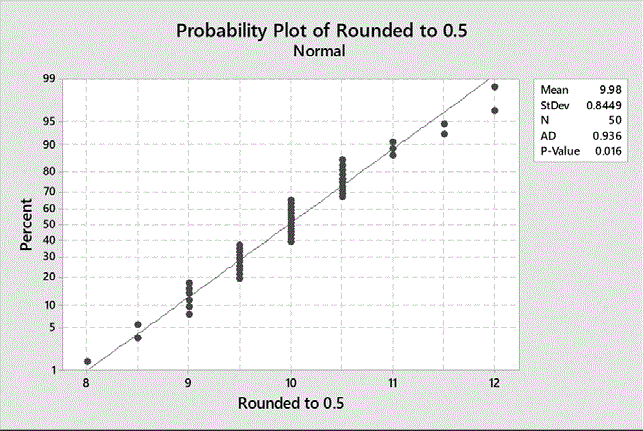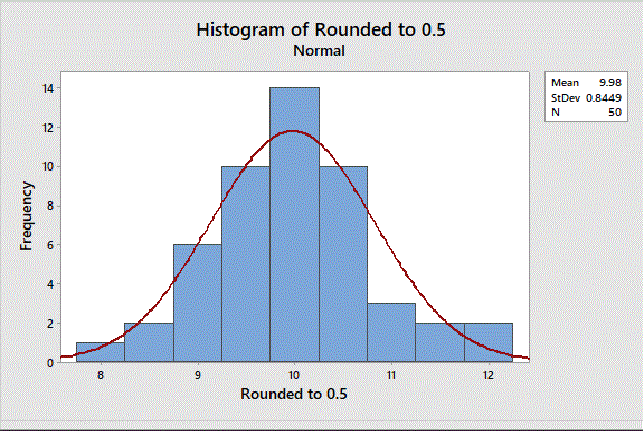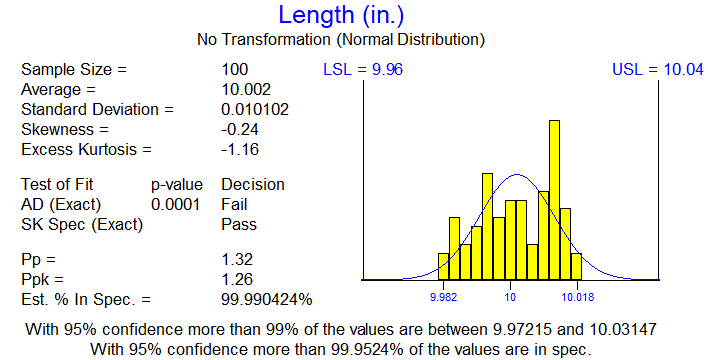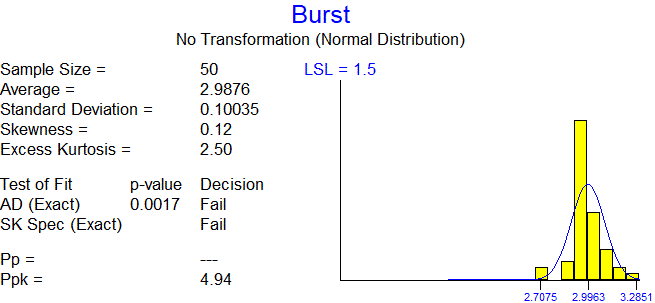# STAT-18: Statistical Techniques for Normality Testing and Transformations

This is part of a series of articles covering the procedures in the book Statistical Procedures for the Medical Device Industry.

### Purpose

To provide guidance on normality testing to ensure the assumption of normality is adequately met when using variables sampling plans and related procedures. Related procedures include normal tolerance intervals, variables confidence limits for the proportion, and confidence statements for Ppk.  Included are procedures to handle situations when the normality test fails and procedures for the detection and handling of outliers.

### Appendices

1. General Normality Tests
2. Effects of Ties on General Normality Tests
3. Skewness–Kurtosis Specific Normality Test
4. Transforming Data
5. Sublotting Data using ANOVA
6. Sublotting Data using Kruskal–Wallis Test
7. Investigating Outliers
8. Invalidating an Outlier Value by Repeated Retesting
9. High Capability Acceptance Criteria

### Highlights

Banding Pattern Due to Ties:  If you see a normal probability plot that looks like that below, the bands are caused by the same value being repeated multiple times (ties).  When this happens both the Anderson-Darling and Shapiro-Wilk test will falsely reject normal data (p-value = 0.016).  The histogram of the data certainly looks normal.  Appendix B describes this problem.  The solution is to use the SK All (D’Agostino–Pearson) test, which is robust to ties in the data.  The SK All test is described in Appendix A and available in the validated spreadsheet STAT-18 – Skewness-Kurtosis Normality Tests accompanying the book.• Bounded by the Normal Distribution:  Not all departures from normality invalidate the use of a variables sampling plan.  The data below fails the general normality tests due to short tails (Anderson-Darling p-value 0.0001).  The data has good capability and seems to be bounded by the normal distribution.  The only thing keeping it from passing is the failed normality test.  The SK Specific test has been designed for this purpose.  It asks the question “Can a variables sampling plan be used?” rather than “Is the data normal?”  It accepts certain departures from normality that do not invalidate the confidence statement associated with the variables sampling plan.  The SK Specific test passes this data allowing the study to pass  The SK Specific test is described in Appendix C and available in the validated spreadsheet STAT-18 – Skewness-Kurtosis Normality Tests accompanying the book.• High Capability Data:  When data has high capability, a normality test may not be required.  Suppose the desired confidence statement is 95%/99% and the plan n=50, Ppk=0.96 was selected.  The data below fails the normality test.  However, since the estimated Ppk is more than 1.84 times the acceptance criteria of 0.96 and the skewness is greater than -2, the high capability acceptance criteria are meet and no normality test is required.  The high capability acceptance criteria are described in Appendix I• Flowchart:  The procedure provides numerous options for handling nonormal data including transforming (Appendix D), sublotting (Appendices E, F), and invalidating outliers (Appendices G, H).  It provides a step-by-step flowchart for deciding which approach to use when.  The flowchart and instructions are important in avoiding the abuse of the these methods to prevent “analysis until it passes”.

### 6 thoughts on “STAT-18: Statistical Techniques for Normality Testing and Transformations”

1.Hello, regarding the high capability data. I was reviewing your book in section STAT-18, Appendix I about this topic. The multiplier table provides multipliers for different values of reliability and sample size.
1) My first question is can I use this to justify not doing normality test before conducting normal tolerance interval analysis? (where PpK = K-factor/3)
2) Secondly, does it matter what confidence I will be testing to in my normal tolerance interval analysis? The examples your provide are for 95% confidence and 99% reliability. Can I also use this table if I plan on doing normal tolerance interval testing on 99% confidence and 99% reliability to justify not having to do normality test?

Thank you.

1.The high capability acceptance criteria also apply to Normal Tolerance Intervals. Since k=3 Ppk, apply the multiplier to the k-value. The table is specific 95% confidence. The multipliers would be somewhat higher for 99% confidence and lower for 90% confidence. The table gives multipliers for different conformance levels and sample sizes. Interpolation between table values is permitted.

2.Hello Wayne,
would it be reasonable to apply the high capability acceptance criteria on data from an unstable process? E.g. expressed by major shifts in mean and variation on samples from multiple batches?

Thank you.

1.Applying variables sampling plans when there is sizeable between lot variation is covered in Appendix C of STAT-03, Statistical Techniques for Process Validation, of my book Statistical Procedures for the Medical Device Industry. When the Between Lot standard deviation is less than 30% of the Total standard deviation, the lots are sufficiently similar that the data can be pooled with no modifications to the procedure. This includes the high capability acceptance criteria. This can be determined using a Variance Components Analysis. All points inside the control chart limits on a control chart is also sufficient evidence.

When between lot variation is greater than 30%, the effective sample size is reduced. For example, suppose there are 3 lots with 10 samples each. Then the total sample size is 30. Depending on the between lot variation, the effective sample size can vary between 30 and 3. Suppose the 10 samples from each lot are identical, meaning there is zero within lot variation but that each lot has a different value. The only variation is between lot variation. In this case, the effective sample size is 3. When there is sizeable between lot variation, the analysis and resulting confidence statements should be based on the effective sample size. It may be necessary to increase the number of lots and the number of samples per lot.

3.Hi – I was just wondering what is the statistical background/ rationale for why the “High Capability Data” is an acceptable approach?

Thank you!

1.The high capability acceptance criteria are explained on pages 584-586 on my book of Statistical Procedures. There it is demonstrated that lack of normality can greatly affect the confidence statement associated with a variables sampling plan. The high capability acceptance criteria are based on Chebyshev’s inequality. This inequality states there are limits to the number of units that can be more than 3, 4, 5 … standard deviations from the mean, regardless of the distribution. This means that if Ppk is high enough, the desired confidence statement can be made regardless of the distribution, so passing a normality test is no longer required. The problem with applying Chebyshev’s inequality is that it is based on the true mean and standard deviation and not estimates. The table of multipliers was developed through extensive simulations to determine how much the Ppk must be increased. I determined that without putting restrictions on the estimated skewness, this approach would not work in practice. I selected the restriction that the estimated skewness be between -2 and 2, because it is generally met. However, generating multipliers for other restrictions on the skewness is possible. Generating the set of multipliers provided took 5 machines 6 months to perform.

Scroll to Top